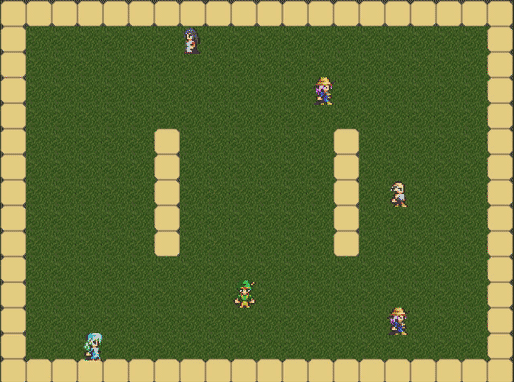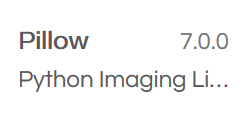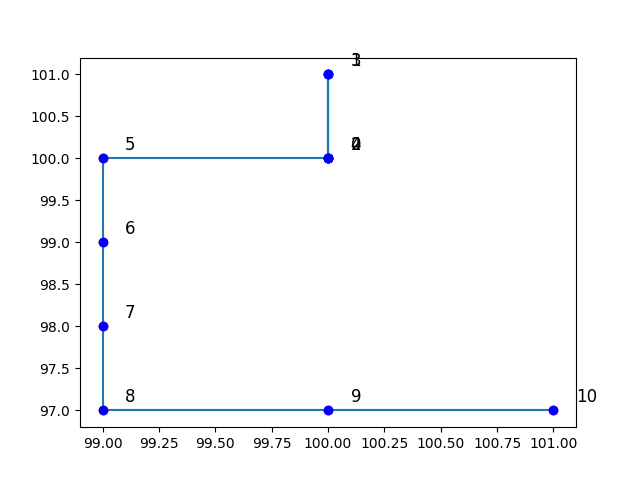# whydoitweet

## Small Sims (1): Simulating a Random WalkIn the first edition of this Small Sims series, I will keep it simple and show you how you can code a random walk. Random Walks are useful in simulation because you can use them to mimic the movements of people wandering around town, for example in games such as these:Source: *http://kidscancode.org/blog/2018/01/godot3_inheritance/*, a site with some great game tutorials by the way.

Another thing you can do with random walks is tocreate cool looking fractal-like images such as these:Image and header image courtesy of László Németh.

Our platform of choice is repl.it, because it allows you all to make and run the code without installing any Python. The platform does have a few glitches though, but I'll carefully highlight them as part of this post.

So our mission is:

• to make a simple simulation that can make a jittery curve like this, reproducing a typical crypto price curve.

In this post I will explain you a way how to make this, and you can follow it in two ways:

1. If you want to follow it as an actual tutorial, then create a new repl.it, and add code to your main.py file whenever you see blocks like:

`#This is an example comment.`

2. Or, you can simply read/skim/browse the post, and download a working repl.it example at the end, which you can then test and modify as you see fit :).

## Creating a repl.it

For full details on how to use repl.it, see the website. However, in a nutshell if you want to create a new “repl”, you can go to the website and press the “new repl” button. You then get a screen like this:...where you can select Python as your programming language. Once you've done that you're all set up, and you'll have a main.py file opened, where you can put in the code from our tutorial. By the way, to run the file, just press the “run >” button at the top.

## Update 05-01-2020: install Pillow as well

Never versions of repl.it don't come with Pillow set up, but you can easily install this inside your repl.it. Just click on the Package Icon on the left side of your screen when you have you repl.it open:And then select the Pillow 7.0.0 package, and press + (once you've pressed it, it will change to a -## What libraries do we need today?

We need to generate random numbers:

`import random`

and we need matplotlib to plot basic data:

`import matplotlib.pyplot as plt`

`import matplotlib as mpl`

`mpl.use('Agg')`

So be sure to put those 4 lines at the top of your main.py. Note: the last two lines are specific to repl.it: they're needed to make sure you can write images without any errors.

## The Random Walk algorithm

So what does the algorithm look like in a very basic form?

As a starting point you have an object (e.g., an NPC) on a given location.

Then, at every time step the object:

- picks a random direction (North, South, East or West)

- and moves one step in that direction.

In code, a function that picks a direction and takes a step will look roughly like this:

`def random_step(xs, ys):`

`"""`

`generate a random step`

`xs: x coordinates of previous locations of the object.`

`ys: y coordinates of previous locations of the object.`

`"""`

`direction = random.randint(0,3)`

`newx = xs[-1]`

`newy = ys[-1]`

`if direction == 0: # Step west`

`newx -= 1`

`if direction == 1: # Step east`

`newx += 1`

`if direction == 2: # Step south`

`newy -= 1`

`if direction == 3: # Step north`

`newy += 1`

`xs.append(newx)`

`ys.append(newy)`

This function actually does most of the work, as you will see.

## Doing an actual Random Walk, using the algorithm

With a function in place to do a single random step, doing a walk is a matter of simply repeating the function many times. For instance, you can do it using a function like this:

`def random_walk(x_start, y_start, steps=10):`

`xswalk = [x_start]`

`yswalk = [y_start]`

`for i in range(0,steps):`

`random_step(xswalk, yswalk)`

`return xswalk,yswalk`

If you'd like to try out if your random walk code works, you can simply run the random_walk function and print the coordinates so you can check them:

`xswalk,yswalk = random_walk(100,100)`

`print(xswalk,yswalk)`

And that's all there is to it! Although... you may want to actually see what your random walk looks like, right?

## Plotting the output of your simulation

To make a graph of your random walk, we will use the commonly used matplotlib library. We'd like to draw lines of the steps taken, points where the object was at each time step, and also numbers next to the points indicating where the object was at each of these steps. Here's a function that covers all three of them:

`def plot_random_walk(xswalk, yswalk, name='random_walk.png'):`

`plt.clf()`

`#plot the lines`

`plt.plot(xswalk, yswalk)`

`#plot the points`

`plt.plot(xswalk, yswalk, 'ro')`

`# add a step number near each point.`

`for i in range(len(xswalk)):`

`x = xswalk[i]`

`y = yswalk[i]`

`plt.plot(x, y, 'bo')`

`plt.text(x + 0.1, y + 0.1 , i, fontsize=12)`

`plt.show()`

`plt.savefig(name)`

...and you can test the plotting by simply calling it again:

`plot_random_walk(xswalk,yswalk)`

NOTE: around this part there are a few glitches in repl.it that are worth noting:

• First, plt.show() doesn't quite work properly in repl.it, but will show your plot on the screen if you run it with a regular Python installation. However, it does save a figure under the name random_walk.png, which you can open on the left side.
• Second, there is a peculiar glitch where the png file is not generated if it already exists. So if you choose to rerun the code, please make sure you delete the random_walk.png file first!

Anyway, once you've done that you'll get a random walk! Mine happens to look like this:As you can see, my agent started at [100,100], and then went North, South, North, South, West, South, South, South, East and East :).

## Closing thoughts

Anyway, all basic stuff but all beginning is simple. Moreover, we'll be using some of this code in future editions in this series, as the Random Walk algorithm is an excellent foundation for more exciting simulations!

So what still remains? Well, I'll leave you with:

1. a question for the experts.
2. an overview of the full code (and a link to the repl I made).
3. (only for Coil subscribers): my feedback to Coil, based on my experience of making this blog post.

So, here we go!

Next: Crypto Price Chart.

## Appendix 1: Expert question

Here's one question to challenge the experts:

• What is the probability that any 10-step random walk does not contain the same location twice?

## Appendix 2: Code summary

Just the full code will look roughly like this:

`import random`

`import matplotlib.pyplot as plt`

`import matplotlib as mpl`

`mpl.use('Agg')`

`# define a function that takes one step in a random direction`

`# we assume 4 possible directions: N,E,S,W.`

`def random_step(xs, ys):`

`direction = random.randint(0,3)`

`newx = xs[-1]`

`newy = ys[-1]`

`if direction == 0:`

`newx -= 1`

`if direction == 1:`

`newx += 1`

`if direction == 2:`

`newy -= 1`

`if direction == 3:`

`newy += 1`

`xs.append(newx)`

`ys.append(newy)`

`def random_walk(x_start, y_start, steps=10):`

`xswalk = [x_start]`

`yswalk = [y_start]`

`for i in range(0,steps):`

`random_step(xswalk, yswalk)`

`return xswalk,yswalk`

`# Let's try it out!`

`xswalk,yswalk = random_walk(100,100)`

`print(xswalk,yswalk)`

`def plot_random_walk(xswalk, yswalk, name='random_walk.png'):`

`plt.clf()`

`#plot the lines`

`plt.plot(xswalk, yswalk)`

`#plot the points`

`plt.plot(xswalk, yswalk, 'ro')`

`# add a step number near each point.`

`for i in range(len(xswalk)):`

`x = xswalk[i]`

`y = yswalk[i]`

`plt.plot(x, y, 'bo')`

`plt.text(x + 0.1, y + 0.1 , i, fontsize=12)`

`plt.show()`

`plt.savefig(name)`

`# Let's try to plot our random walk`

`plot_random_walk(xswalk,yswalk)`

And a working repl.it of it can be found here.

If you have any specific questions or comments, feel free to contact me by e-mail (djgroennl@gmail.com) or via Twitter (@whydoitweet).

Continue reading with a Coil membership.